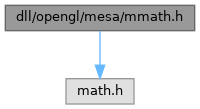ReactOS  0.4.15-dev-3451-gf606fec
mmath.h File Reference
`#include <math.h>`
Include dependency graph for mmath.h:This graph shows which files directly or indirectly include this file:Go to the source code of this file.

## Macros

#define FloatToInt(F)   lrintf((F))

#define GL_SQRT(X)   sqrt(X)

#define NORMALIZE_3FV(V)

## Functions

float gl_sqrt (float x)

void gl_init_math (void)

## ◆ FloatToInt

 #define FloatToInt ( F ) lrintf((F))

Definition at line 56 of file mmath.h.

## ◆ GL_SQRT

 #define GL_SQRT ( X ) sqrt(X)

Definition at line 63 of file mmath.h.

## ◆ NORMALIZE_3FV

 #define NORMALIZE_3FV ( V )
Value:
{ \
GLfloat len; \
len = GL_SQRT(V*V+V*V+V*V); \
if (len>0.0001F) { \
len = 1.0F / len; \
V *= len; \
V *= len; \
V *= len; \
} \
}
#define GL_SQRT(X)
Definition: mmath.h:63
GLenum GLsizei len
Definition: glext.h:6722
#define F(x, y, z)
Definition: md5.c:51

Definition at line 68 of file mmath.h.

## ◆ gl_init_math()

 void gl_init_math ( void )

Definition at line 140 of file mmath.c.

141 {
142  static GLboolean initialized = GL_FALSE;
143
144  if (!initialized) {
145  init_sqrt();
146
147
149  }
150 }
#define GL_FALSE
Definition: gl.h:173
unsigned char GLboolean
Definition: gl.h:151
static BOOL initialized
Definition: syslog.c:39
#define GL_TRUE
Definition: gl.h:174
static void init_sqrt(void)
Definition: mmath.c:63

Referenced by gl_create_context().

## ◆ gl_sqrt()

 float gl_sqrt ( float x )

Definition at line 104 of file mmath.c.

105 {
106 #ifdef FAST_MATH
107  unsigned int *num = (unsigned int *)&x;
108  /* to access the bits of a float in C
109  * we must misuse pointers */
110
111  short e; /* the exponent */
112  if (x == 0.0F) return 0.0F; /* check for square root of 0 */
113  e = (*num >> 23) - 127; /* get the exponent - on a SPARC the */
114  /* exponent is stored with 127 added */
115  *num &= 0x7fffff; /* leave only the mantissa */
116  if (e & 0x01) *num |= 0x800000;
117  /* the exponent is odd so we have to */
118  /* look it up in the second half of */
119  /* the lookup table, so we set the */
120  /* high bit */
121  e >>= 1; /* divide the exponent by two */
122  /* note that in C the shift */
123  /* operators are sign preserving */
124  /* for signed operands */
125  /* Do the table lookup, based on the quaternary mantissa,
126  * then reconstruct the result back into a float
127  */
128  *num = ((sqrttab[*num >> 16]) << 16) | ((e + 127) << 23);
129  return x;
130 #else
131  return sqrt(x);
132 #endif
133 }
_STLP_DECLSPEC complex< float > _STLP_CALL sqrt(const complex< float > &)
Definition: complex.cpp:188
GLint GLint GLint GLint GLint x
Definition: gl.h:1548
#define e
Definition: ke_i.h:82
static short sqrttab[0x100]
Definition: mmath.c:61
GLuint GLuint num
Definition: glext.h:9618
#define F(x, y, z)
Definition: md5.c:51